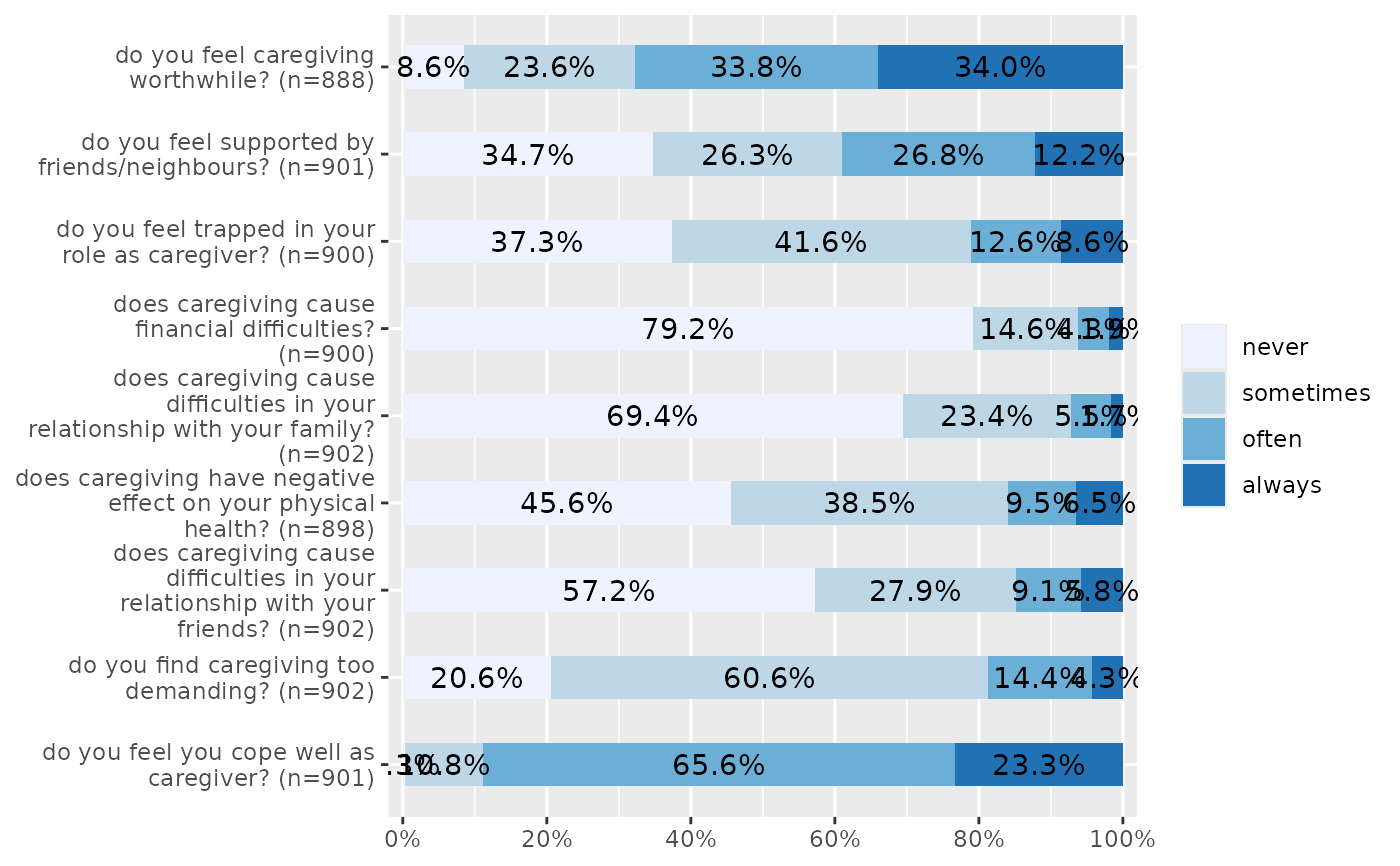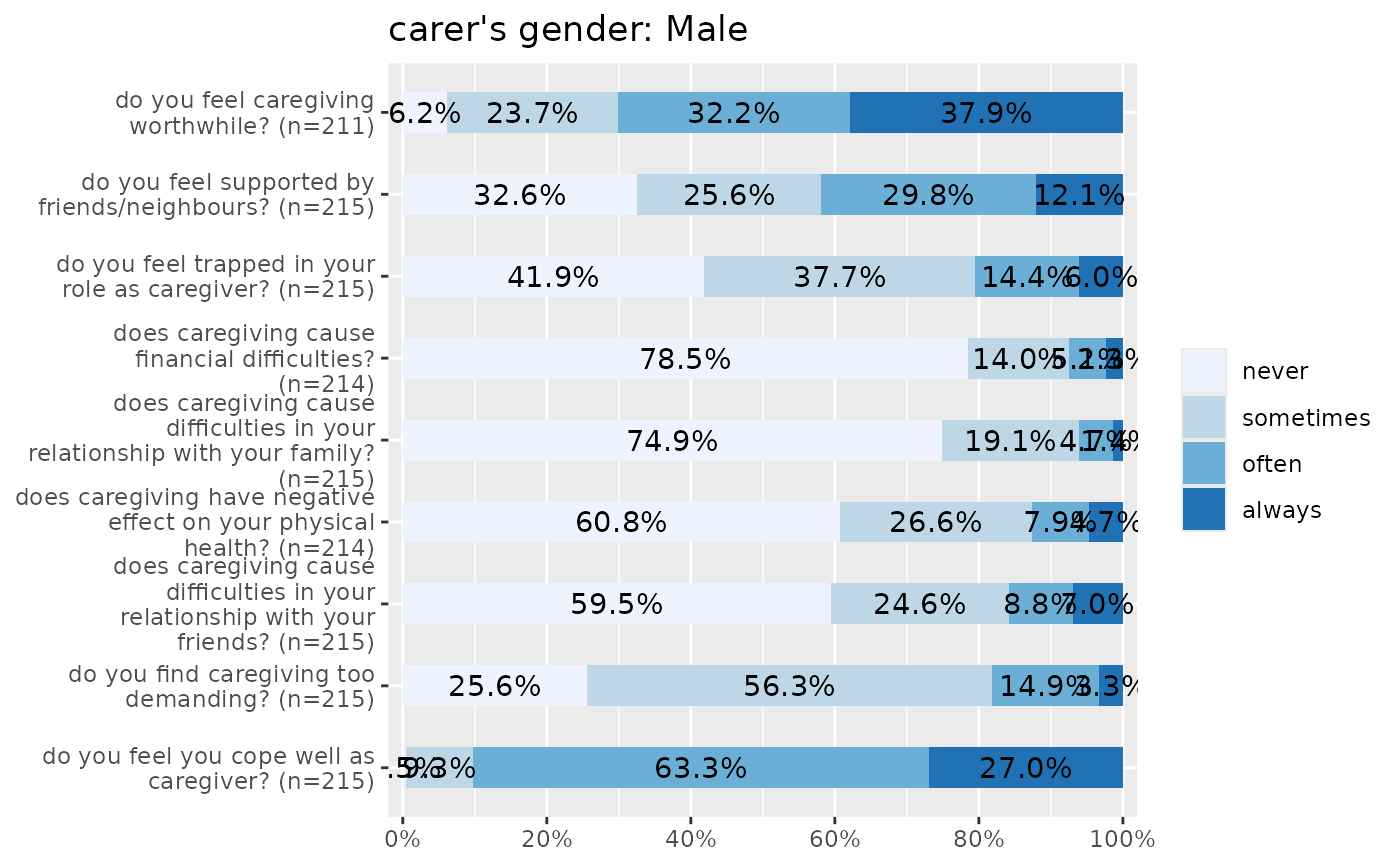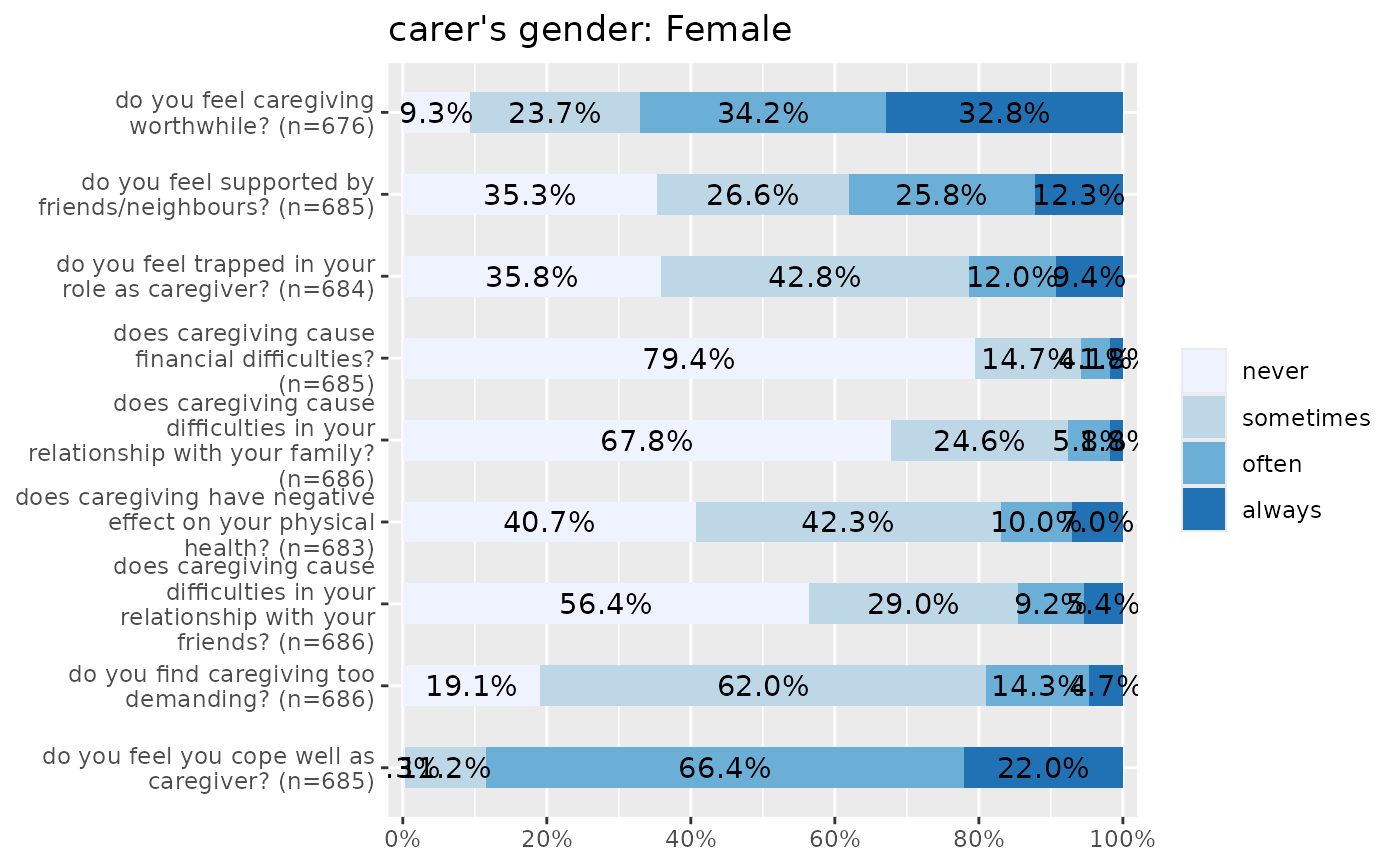Plot items (variables) of a scale as stacked proportional bars. This function is useful when several items with identical scale/categoroies should be plotted to compare the distribution of answers.

plot_stackfrq(
items,
title = NULL,
legend.title = NULL,
legend.labels = NULL,
axis.titles = NULL,
axis.labels = NULL,
weight.by = NULL,
sort.frq = NULL,
wrap.title = 50,
wrap.labels = 30,
wrap.legend.title = 30,
wrap.legend.labels = 28,
geom.size = 0.5,
geom.colors = "Blues",
show.prc = TRUE,
show.n = FALSE,
show.total = TRUE,
show.axis.prc = TRUE,
show.legend = TRUE,
grid.breaks = 0.2,
expand.grid = FALSE,
digits = 1,
vjust = "center",
coord.flip = TRUE
)

Arguments

items

Data frame, or a grouped data frame, with each column representing one item.

title

character vector, used as plot title. Depending on plot type and function, will be set automatically. If title = "", no title is printed. For effect-plots, may also be a character vector of length > 1, to define titles for each sub-plot or facet.

legend.title

character vector, used as title for the plot legend.

legend.labels

character vector with labels for the guide/legend.

axis.titles

character vector of length one or two, defining the title(s) for the x-axis and y-axis.

axis.labels

character vector with labels used as axis labels. Optional argument, since in most cases, axis labels are set automatically.

weight.by

Vector of weights that will be applied to weight all cases. Must be a vector of same length as the input vector. Default is NULL, so no weights are used.

sort.frq

Indicates whether the items should be ordered by by highest count of first or last category of items.

"first.asc"

to order ascending by lowest count of first category,

"first.desc"

to order descending by lowest count of first category,

"last.asc"

to order ascending by lowest count of last category,

"last.desc"

to order descending by lowest count of last category,

NULL

(default) for no sorting.

wrap.title

numeric, determines how many chars of the plot title are displayed in one line and when a line break is inserted.

wrap.labels

numeric, determines how many chars of the value, variable or axis labels are displayed in one line and when a line break is inserted.

wrap.legend.title

numeric, determines how many chars of the legend's title are displayed in one line and when a line break is inserted.

wrap.legend.labels

numeric, determines how many chars of the legend labels are displayed in one line and when a line break is inserted.

geom.size

size resp. width of the geoms (bar width, line thickness or point size, depending on plot type and function). Note that bar and bin widths mostly need smaller values than dot sizes.

geom.colors

user defined color for geoms. See 'Details' in plot_grpfrq.

show.prc

Logical, whether percentage values should be plotted or not.

show.n

Logical, whether count values hould be plotted or not.

show.total

logical, if TRUE, adds total number of cases for each group or category to the labels.

show.axis.prc

Logical, if TRUE (default), the percentage values at the x-axis are shown.

show.legend

logical, if TRUE, and depending on plot type and function, a legend is added to the plot.

grid.breaks

numeric; sets the distance between breaks for the axis, i.e. at every grid.breaks'th position a major grid is being printed.

expand.grid

logical, if TRUE, the plot grid is expanded, i.e. there is a small margin between axes and plotting region. Default is FALSE.

digits

Numeric, amount of digits after decimal point when rounding estimates or values.

vjust

character vector, indicating the vertical position of value labels. Allowed are same values as for vjust aesthetics from ggplot2: "left", "center", "right", "bottom", "middle", "top" and new options like "inward" and "outward", which align text towards and away from the center of the plot respectively.

coord.flip

logical, if TRUE, the x and y axis are swapped.

A ggplot-object.

Examples

# Data from the EUROFAMCARE sample dataset

data(efc)
# recveive first item of COPE-index scale
start <- which(colnames(efc) == "c82cop1")
# recveive first item of COPE-index scale
end <- which(colnames(efc) == "c90cop9")
# auto-detection of labels
plot_stackfrq(efc[, start:end])# works on grouped data frames as well

efc %>%
group_by(c161sex) %>%
select(start:end) %>%
plot_stackfrq()
#> Note: Using an external vector in selections is ambiguous.
#>  Use all_of(start) instead of start to silence this message.
#>  See <https://tidyselect.r-lib.org/reference/faq-external-vector.html>.
#> This message is displayed once per session.
#> Note: Using an external vector in selections is ambiguous.
#>  Use all_of(end) instead of end to silence this message.
#>  See <https://tidyselect.r-lib.org/reference/faq-external-vector.html>.
#> This message is displayed once per session.
#> Adding missing grouping variables: c161sex
#> []
#> [][]
#> NULL
#>
#> [][]#>
#>
#> []#>